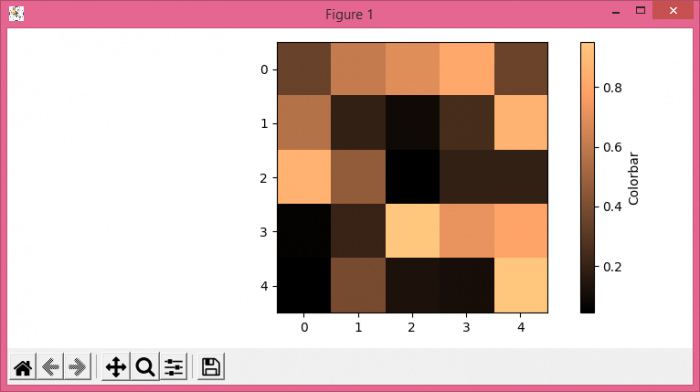# How to give Matplolib imshow plot colorbars a label?

To give matplotlib imshow() plot colorbars a label, we can take the following steps −

• Set the figure size and adjust the padding between and around the subplots.

• Create 5×5 data points using Numpy.

• Use imshow() method to display the data as an image, i.e., on a 2D regular raster.

• Create a colorbar for a ScalarMappable instance, im.

• Set colorbar label using set_label() method.

• To display the figure, use show() method.

## Example

import numpy as np
from matplotlib import pyplot as plt

plt.rcParams["figure.figsize"] = [7.50, 3.50]
plt.rcParams["figure.autolayout"] = True

data = np.random.rand(5, 5)

im = plt.imshow(data, cmap="copper")

cbar = plt.colorbar(im)
cbar.set_label("Colorbar")

plt.show()

## Output